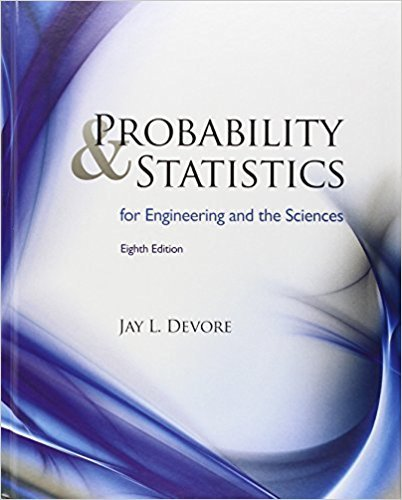×
×

# Solutions for Chapter 14: Goodness-of-Fit Tests and Categorical Data Analysis## Full solutions for Probability and Statistics for Engineering and the Sciences | 8th Edition

ISBN: 9780538733526Solutions for Chapter 14: Goodness-of-Fit Tests and Categorical Data Analysis

Solutions for Chapter 14
4 5 0 401 Reviews
17
3
##### ISBN: 9780538733526

Chapter 14: Goodness-of-Fit Tests and Categorical Data Analysis includes 49 full step-by-step solutions. This textbook survival guide was created for the textbook: Probability and Statistics for Engineering and the Sciences , edition: 8. This expansive textbook survival guide covers the following chapters and their solutions. Since 49 problems in chapter 14: Goodness-of-Fit Tests and Categorical Data Analysis have been answered, more than 28888 students have viewed full step-by-step solutions from this chapter. Probability and Statistics for Engineering and the Sciences was written by and is associated to the ISBN: 9780538733526.

Key Statistics Terms and definitions covered in this textbook
• Alias

In a fractional factorial experiment when certain factor effects cannot be estimated uniquely, they are said to be aliased.

• Central tendency

The tendency of data to cluster around some value. Central tendency is usually expressed by a measure of location such as the mean, median, or mode.

• Coeficient of determination

See R 2 .

• Combination.

A subset selected without replacement from a set used to determine the number of outcomes in events and sample spaces.

• Components of variance

The individual components of the total variance that are attributable to speciic sources. This usually refers to the individual variance components arising from a random or mixed model analysis of variance.

• Conditional probability distribution

The distribution of a random variable given that the random experiment produces an outcome in an event. The given event might specify values for one or more other random variables

• Conidence coeficient

The probability 1?a associated with a conidence interval expressing the probability that the stated interval will contain the true parameter value.

• Critical region

In hypothesis testing, this is the portion of the sample space of a test statistic that will lead to rejection of the null hypothesis.

• Defect concentration diagram

A quality tool that graphically shows the location of defects on a part or in a process.

• Design matrix

A matrix that provides the tests that are to be conducted in an experiment.

• Discrete distribution

A probability distribution for a discrete random variable

• Discrete random variable

A random variable with a inite (or countably ininite) range.

• Dispersion

The amount of variability exhibited by data

• Error propagation

An analysis of how the variance of the random variable that represents that output of a system depends on the variances of the inputs. A formula exists when the output is a linear function of the inputs and the formula is simpliied if the inputs are assumed to be independent.

• Experiment

A series of tests in which changes are made to the system under study

• F-test

Any test of signiicance involving the F distribution. The most common F-tests are (1) testing hypotheses about the variances or standard deviations of two independent normal distributions, (2) testing hypotheses about treatment means or variance components in the analysis of variance, and (3) testing signiicance of regression or tests on subsets of parameters in a regression model.

• First-order model

A model that contains only irstorder terms. For example, the irst-order response surface model in two variables is y xx = + ?? ? ? 0 11 2 2 + + . A irst-order model is also called a main effects model

• Fixed factor (or fixed effect).

In analysis of variance, a factor or effect is considered ixed if all the levels of interest for that factor are included in the experiment. Conclusions are then valid about this set of levels only, although when the factor is quantitative, it is customary to it a model to the data for interpolating between these levels.

• Forward selection

A method of variable selection in regression, where variables are inserted one at a time into the model until no other variables that contribute signiicantly to the model can be found.

• Fraction defective control chart

See P chart

×